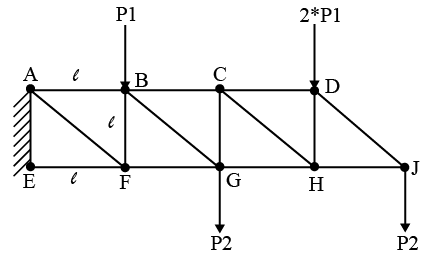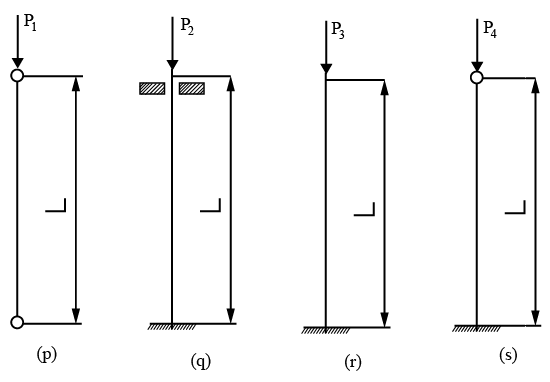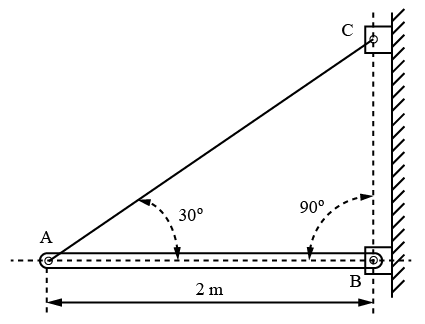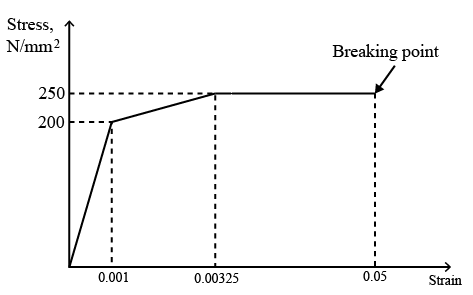# ISRO Scientist or Engineer Mechanical Dec 2017

Instructions

For the following questions answer them individually

Question 1

# The most applicable failure theory for metals like Aluminium isQuestion 2

# Which one of the following is FALSE?Question 3

# A statistical process control chart which shows the number of defects found in a subgroup of fixed size isQuestion 4

# Complex irregular three dimensional surfaces can be located using the following methodQuestion 5

# The force in member BC of steel truss structure shown in figure below is (Cross section of each member is A, Length of each member is ‘l')Question 6

# Buckling loads (Pi) of 4 columns of equal length and cross section, but with different end conditions are shown below. Which ofthe following is TRUE?Question 7

# The bar AB weighs 10 kg per meter and is supported by cable AC and a pin at B. Reaction at B is ( take g = 10 m/s$$^2$$)Question 8

# Arrange the metals Aluminium, Copper, Iron & Silver in increasing order of thermal conductivityQuestion 9

# Modulus of resilience for the below material isQuestion 10

# Which of the following is FALSE?OR